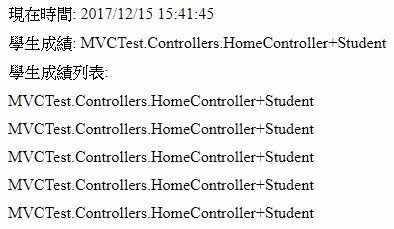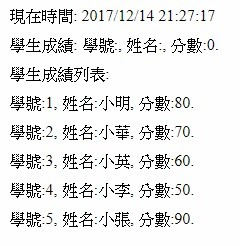#2018 iT 邦幫忙鐵人賽DAY 6
0
Modern Web

## [Day 06] ASP.NET MVC後端傳資料到前端介紹(二)

``````public class Student
{
public string id { get; set; }
public string name { get; set; }
public int score { get; set; }
public Student()
{
id = string.Empty;
name = string.Empty;
score = 0;
}
public Student(string _id, string _name, int _score)
{
id = _id;
name = _name;
score = _score;
}
public override string ToString()
{
return \$"學號:{id}, 姓名:{name}, 分數:{score}.";
}
}
``````

``````public Student()
{
id = string.Empty;
name = string.Empty;
score = 0;
}
``````

``````public Student(string _id, string _name, int _score)
{
id = _id;
name = _name;
score = _score;
}
``````

``````Student student = new Student();
student.id = "1";
student.name = "小明";
student.score = 80;
``````

``````Student student = new Student("1", "小明", 80);
``````

``````public override string ToString()
{
return \$"學號:{id}, 姓名:{name}, 分數:{score}.";
}
``````